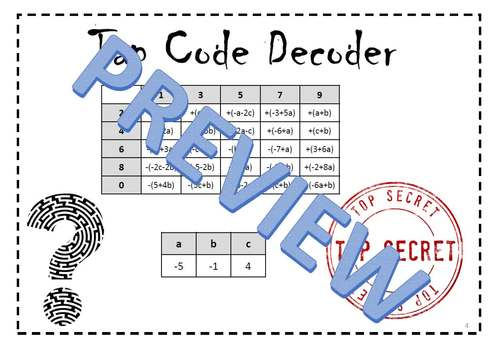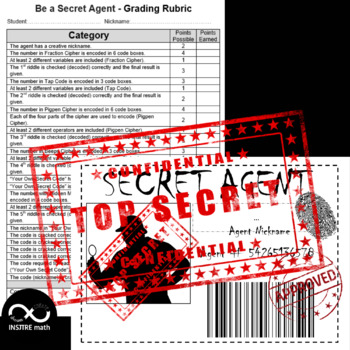# Algebra Project Based Learning Algebraic Expressions Equations Be a Secret Agent

Rated 4.67 out of 5, based on 6 reviews
6 Ratings;
6th - 9th, Homeschool
Subjects
Resource Type
Formats Included
• PDF
•Google Apps™
Pages
19 pages
Report this resource to TPTThe Teacher-Author indicated this resource includes assets from Google Workspace (e.g. docs, slides, etc.).
##### Also included in
1. This is a perfect end of the year, back to school, math centers, summer camp, or summer school Math Bundle (evaluating algebraic expressions, simplify algebraic expressions by combining like terms and using the distributive property, and solving one and two-step equations):1. Treasure Hunt - Simplif
Price \$10.99Original Price \$13.98Save \$2.99
2. This is a perfect end of the year, back to school, math centers, summer camp, or summer school 7th, 8th, 9th grade Math Bundle (mean, median, mode, range, circumference &amp; area of a circle, coordinate plane, evaluating algebraic expressions, simplify algebraic expressions by combining like terms
Price \$11.50Original Price \$14.48Save \$2.98
3. This is a perfect end of the year, back to school, math centers, summer camp, or summer school math bundle (Project Based Learning - multi-digit addition, subtraction, and multiplication (including decimals), money management, area and perimeter calculation; Percentages, Mean, Median, Mode, Range; P
Price \$11.90Original Price \$14.97Save \$3.07
4. This is a perfect end of the year, back to school, math centers, summer camp, or summer school math bundle to practice fraction and mixed numbers operations, multi-digit addition, subtraction, division, and multiplication (including decimals), money management, area and perimeter calculation, percen
Price \$15.99Original Price \$20.97Save \$4.98
5. This is a perfect back to school, first days of school, end of the year, math centers, summer camp, or summer school math bundle to practice fraction and mixed numbers operations, multi-digit addition, subtraction, division, and multiplication (including decimals), money management, area and perimet
Price \$16.99Original Price \$20.96Save \$3.97
6. This is a perfect back to school, first days of school, end of the year, math centers, summer camp, or summer school math bundle to practice fraction and mixed numbers operations, multi-digit addition, subtraction, division, and multiplication (including decimals), money management, area and perimet
Price \$16.10Original Price \$20.16Save \$4.06
7. MATH PBL Middle School BUNDLE. This is a perfect back to school, first days of school, end of the year, math centers, summer camp, or summer school math bundle to practice fraction and mixed numbers operations, multi-digit addition, subtraction, division, and multiplication (including decimals), mon
Price \$18.99Original Price \$24.15Save \$5.16
8. This is a perfect Fall and Thanksgiving Math bundle to practice evaluating algebraic expressions, simplify algebraic expressions by combining like terms and using the distributive property, and solving One-Step, Two-Step, and Multi-Step Equations.1. Be a Secret Agent - Algebra Project Based Learnin
Price \$12.99Original Price \$15.96Save \$2.97
9. This is a perfect Fall Math bundle to practice finding the slope and y-intercept, graphing linear equations, laws of exponents, evaluating algebraic expressions, simplify algebraic expressions by combining like terms and using the distributive property, and solving one-step, two-step, and multi-step
Price \$11.19Original Price \$13.98Save \$2.79
10. This is a perfect Enrichment bundle to practice fraction and mixed numbers operations, multi-digit addition, subtraction, division, and multiplication (including decimals), money management, area and perimeter calculation, algebraic expressions &amp; equations, unit price calculation, percentages, o
Price \$36.99Original Price \$49.29Save \$12.30
11. Full Year Bundle - 16 Fun Math PBL Activities. This is a perfect Enrichment bundle to practice fraction &amp; mixed numbers, decimals, percentages, integers, area and perimeter, algebraic expressions &amp; equations, unit price, volume &amp; surface area of prisms and pyramids, ratios &amp; proporti
Price \$44.99Original Price \$64.26Save \$19.27
12. Must-have Math Enrichment Projects (PBL) Bundle: Decimals, Fractions, Percentages, Percents, Integers, Geometry (Area and Perimeter), Unit Rates, Algebraic Expressions, One and Two-Step Equations. This bundle includes my 8 best-selling math projects.Save 30% by purchasing this bundle!If you want you
Price \$25.29Original Price \$36.13Save \$10.84
13. Full Year Bundle. If you want your math lessons to be an unforgettable experience for your students, be sure to check out this BUNDLE.38 Math Resources: Math &amp; Art projects, Project-Based Learning activities, Escape Rooms, Discovery activities, Pixel Art Mystery Pictures, Challenges, and more.S
Price \$72.00Original Price \$110.86Save \$38.86
14. 21 Math Resources: Math &amp; Art projects, Project-Based Learning activities, Escape Rooms, Discovery activities, Pixel Art Mystery Pictures, and more. If you want your math lessons to be an unforgettable experience for your students, be sure to check out this BUNDLE.Save 30% by purchasing this bun
Price \$49.50Original Price \$70.73Save \$21.23

### Description

Math Enrichment Algebra PBL Project - Printable & Digital (EDITABLE). This is a perfect end of the year, back to school, math centers, summer camp, or summer school math activity to practice evaluating algebraic expressions, simplifying algebraic expressions by combining like terms and using the distributive property, and solving one and two-step equations.

Students want to be Secret Agents, so they have to pass an exam in encrypting and decrypting messages. If they pass this test, they will earn a Secret Agent Badge.

Students will:

- encode numbers in 5 different ciphers,

- create their own secret code,

- encode the final message and their nickname in the created code,

- crack codes prepared by their classmate.

This project covers:

- Simplifying Algebraic Expressions (Distributive Property, Combining Like Terms)

- Evaluating Algebraic Expressions

- One-Step Equations

- Two-Step Equations

This resource includes:

- The editable digital version in Google Slides™.

- The editable PowerPoint™ version.

- The printable version in pdf (color).

- The printable version in pdf (black & white).

- An example of a completed project.

Look at the preview to check how it works.

Click the GREEN STAR at the top of my store to follow me and be informed about my new products and sales. (or >> CLICK HERE)

Check out Inspire Math Matematyka for more resources.

Math&Art

Projects Based Learning

Inquiry Based Learning

Back to School/ First Day

End of the Year Activities

Drag & Drop Activities

Digital Escape Rooms

Pixel Art Mysteries

Games

Scavenger Hunts

Treasure Hunts

Total Pages
19 pages